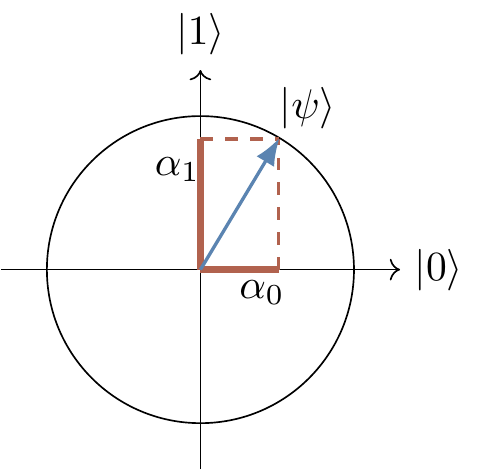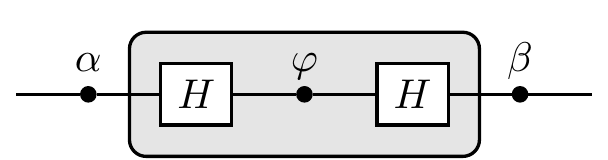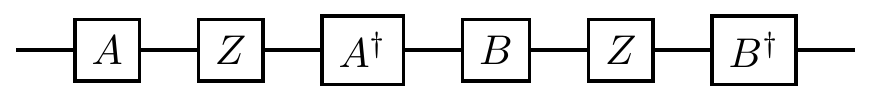## 4.2 Complete measurements

A projector is any Hermitian (P=P^\dagger) operator which is idempotent (P^2=P). The rank of P is given by \operatorname{tr}(P). In the Dirac notation, if |e\rangle is a unit vector, then |e\rangle\langle e| is a rank-one projector on the subspace spanned by |e\rangle, and it acts on any vector |v\rangle via (|e\rangle\langle e|)|v\rangle = |e\rangle\langle e|v\rangle.

The most common measurement in quantum information science is the standard measurement on a qubit, also referred to as the measurement in the standard (or computational) basis: \{|0\rangle,|1\rangle\}. When we draw circuit diagrams it is tacitly assumed that such a measurement is performed on each qubit at the end of quantum evolution.Figure 4.1: The standard/computational basis defines the so-called standard measurements.

However, if we want to emphasise the role of the measurement, then we can include it explicitly in the diagram as a special quantum gate, e.g. asor, in an alternative notation, asAs we can see, if the qubit is prepared in state |\psi\rangle = \alpha_0|0\rangle + \alpha_1|1\rangle and subsequently measured in the standard basis, then the outcome is |k\rangle (for k=0,1) with probability91 \begin{aligned} |\alpha_k|^2 &= |\langle k|\psi\rangle|^2 \\&= \underbrace{\langle\psi|k\rangle}_{\alpha_k^\star} \underbrace{\langle k|\psi\rangle}_{\alpha_k} \\&= \langle\psi| \underbrace{|k\rangle\langle k|}_{\text{projector}} |\psi\rangle \\&= \langle\psi|P_k|\psi\rangle \end{aligned} where P_k=|k\rangle\langle k| is the projector on |k\rangle. If the outcome of the measurement is |k\rangle, then the output state of the measurement gate is |k\rangle. The original state |\psi\rangle is irretrievably lost. This sudden change of the state, from the pre-measurement state |\psi\rangle to the post-measurement state, either |0\rangle or |1\rangle, is often called a collapse or a reduction of the state.

So it looks like there are two distinct ways for a quantum state to change: on the one hand we have unitary evolutions, and on the other hand we have an abrupt change during the measurement process. Surely, the measurement process is not governed by any different laws of physics?

No, it is not!

The subtleties (both mathematical and philosophical) of quantum collapse are still very much active topics of research, and we could spend an entire book discussing them. There are a lot of other sources where you can read about such things — here is a very short list to start:

• T. Norson, Foundations of Quantum Mechanics: An Exploration of the Physical Meaning of Quantum Theory. Springer, 2017. ISBN: 978-3-319-65867-4. DOI: 10.1007/978-3-319-65867-4.
• M. Schlosshauer, “Decoherence, the measurement problem, and interpretations of quantum mechanics”. Rev. Mod. Phys. 76 (2004), pp. 1267–1305. arXiv:quant-ph/0312059.
• F. Giacosa, “On unitary evolution and collapse in Quantum Mechanics”. Quanta 3 (2014), pp. 156–170. arXiv:1406.2344.

A measurement is a physical process and can be explained without any “collapse”, but it is usually a complicated process in which one complex system (a measuring apparatus or an observer) interacts and gets correlated with a physical system being measured. We will discuss this more later on, but for now let us accept a “collapse” as a convenient mathematical shortcut, and describe it in terms of projectors rather than unitary operators.

For our purposes, the idea of “quantum collapse” is simply a way of black boxing the irreversible interaction between a quantum system and its surrounding classical environment.

On a practical level, it means that we describe measurement and observation with projectors instead of unitary operators.

1. This slick argument is a good example of how nice the bra-ket notation can be when we leverage the ambiguity of an expression like \langle a||b\rangle|b\rangle\langle a|, which we can read as the scalar product of two scalars or as a projector sandwiched between a bra and a ket.↩︎